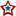#MN-Sen: Recount Regression, Day 1

Using the pretense of my pre-recount audit analysis I've incorporated the initial recount returns into the regression mechanism.

For a more complete explanation of the process used, please refer to my initial post on the subject.

The following two graphs depict the number of votes gained or lost with respect to the total votes recorded for each precinct that has completed their recount. Each dot represents the change between the originally certified result and the post recount tally in a given precinct with x number of total votes:And the current functions used in conjunction with the regression:Now again using those functions, the following two graphs further illustrate the regression interpolation. The graph below illustrates the regression on precincts whose vote totals are less than 2,668; the largest precinct that has completed a recount.The graph below goes further and fits the regression onto all 4,130 precincts statewide; this forces 214,813 additional votes, that lie in precincts that surpass the 2,668 vote threshold, into the predetermined trend.Using the previous two graphs, and the functions they represent, a projection can be made for the cases covered. The first case simply includes precincts with 2,668 or less, while precincts above and beyond that figure are entirely ignored. For each precinct, the total vote total is taken and applied to the listed function for each candidate. The result is then added to that candidate's sum, and the next precinct is calculated. This process is done using precinct results from the final certification.

```            Coleman Gain: -407.600629299817 (+/- 2.4)
Franken Gain: -194.977755790799 (+/- .62)

Franken Net: 212.622873509018 (+/- 3.02)
```

Franken nearly makes up the pre-recount deficit of 215 votes, while 214,813 votes are entirely ignored. If those additional votes are applied to the process, Franken takes a decisive lead:

```           Coleman Gain: -540.336882632741 (+/- 4925)
Franken Gain: -237.764374574729 (+/- 6342)

Franken Net: 302.572508058013 (+/- 11267)
```

As of right now (4:16 AM CT 11/20), after 15.49% of all votes have been recounted, Franken stands poised to become Minnesota's next Senator. Later today, after the second day of recounting is over, and the officially unofficial results are posted at the SOS site, I'll post another article with the new data included in the analysis. As each day goes by, the graphs, and projections should begin to converge. If Franken gains another 40 votes throughout the upcoming day, Team Coleman is definitely in trouble. The only way to find out is by counting the votes.

## 0 Response(s) to MN-Sen: Recount Regression, Day 1(Word Verification)